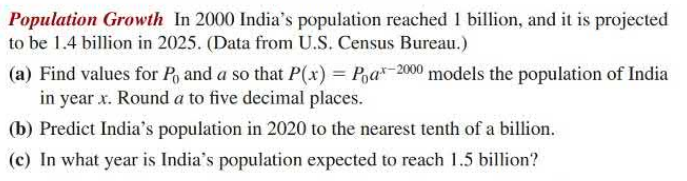### Still have math questions?

Algebra
QuestionPopulation Growth In $$2000$$ India's population reached $$1$$ billion, and it is projected to be $$1.4$$ billion in $$2025$$ . (Data from U.S. Census Bureau.)

(a) Find values for $$P _ { 0 }$$ and $$a$$ so that $$P ( x ) = P _ { 0 } a ^ { x - 2000 }$$ models the population of India in year $$x$$ . Round $$a$$ to five decimal places.

(b) Predict India's population in $$2020$$ to the nearest tenth of a billion.

(c) In what year is India's population expected to reach $$1.5$$ billion?

$$\left. \begin{array} { l l l } { \text { (a) } P _ { 0 } = 1 ; a \approx 1.01355 } & { \text { (b) } 1.3 \text { billion } } & { \text { (c) } 2030 } \end{array} \right.$$